Courses

# Gas Power Cycles - MCQ Test 1

## 20 Questions MCQ Test GATE Mechanical (ME) 2022 Mock Test Series | Gas Power Cycles - MCQ Test 1

Description
This mock test of Gas Power Cycles - MCQ Test 1 for Mechanical Engineering helps you for every Mechanical Engineering entrance exam. This contains 20 Multiple Choice Questions for Mechanical Engineering Gas Power Cycles - MCQ Test 1 (mcq) to study with solutions a complete question bank. The solved questions answers in this Gas Power Cycles - MCQ Test 1 quiz give you a good mix of easy questions and tough questions. Mechanical Engineering students definitely take this Gas Power Cycles - MCQ Test 1 exercise for a better result in the exam. You can find other Gas Power Cycles - MCQ Test 1 extra questions, long questions & short questions for Mechanical Engineering on EduRev as well by searching above.
QUESTION: 1

### A heat transformer is a device that transfers a part of the heat, supplied to itat an intermediate temperature , to a high temperature reservoir while rejecting the remaining part to a low temperature heat sink. In such a heat transformer, 100 kJ of heat is supplied at 350 K. The maximum amount of heatin kJ that can be transferred to 400 K, when the rest is rejected to that can betransferred to 400 K, when rest is rejected to a heat sink at 300 K is

Solution: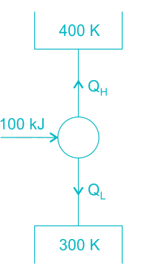​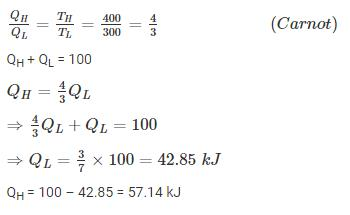QUESTION: 2

### Which one of the following is NOT a necessary assumption for the airstandard Otto cycle?

Solution:

Ans. (b) Intake process isn’t constant volume heat rejection processes. it is
constant pressure process.

QUESTION: 3

###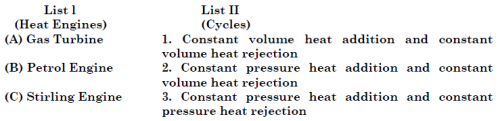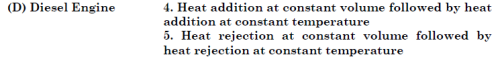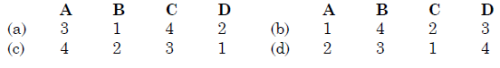Solution:

Ans. (a)

QUESTION: 4

A diesel engine is usually more efficient than a spark ignition engine because

Solution:

Ans (c)

QUESTION: 5

For a heat engine operating on the Carnot cycle, the work output is ¼ th of the heat transferred to the sink. The efficiency of the engine is

Solution:

Q1->heat transferred from source to heat engine

Q2->heat transferred from heat engine to sink

So, q1 = w + q2           --(1)

Given data

w =q2 /4        - (2)

Substitute 2 in 1 you will get
q1 = q2 /4 + q2 * q1 = (5*q2) /4     --(3)

efficiency for heat engine =1-(q2 /q1)

Now substitute 3 in above equation efficiency = 1-(4*q1/(5*q1)) = 1-(4/5)  =1/5 = 0.2*10 =20%

QUESTION: 6

Brayton cycle with infinite inter-cooling and reheating stages wouldapproximate a

Solution:

Ans. (b)

QUESTION: 7

Consider the following statements regarding Otto cycle:
1. It is not a reversible cycle.
2. Its efficiency can be improved by using a working fluid of higher value ofratio of specific heats.
3. The practical way of increasing its efficiency is to increase the compressionratio.
4. Carburetted gasoline engines working on Otto cycle can work withcompression ratios more than
Of these statements

Solution:

Ans. (d)

QUESTION: 8

Consider the following statements:
1. For a Diesel cycle, the thermal efficiency decreases as the cut off ratioincreases.
2. In a petrol engine the high voltage for spark is in the order of 1000 V
3. The material for centre electrode in spark plug is carbon.
Which of the statements given above is/are correct?

Solution:

Ans. (a) 2 is false, the voltage for spark is in the order of 25 KV
3 is false, best material platinum but mostly used nickel alloy.

QUESTION: 9

Assertion (A): The air standard efficiency of the diesel cycle decreases as the load is increased Reason (R): With increase of load, cut-off ratio increases.

Solution:

Ans. (a)

QUESTION: 10

For the same maximum pressure and heat input

Solution:

Ans. (a)

QUESTION: 11

The order of values of thermal efficiency of Otto, Diesel and Dual cycle, when they have equal compression ratio and heat rejection, is given by

Solution:

Ans. (d)

QUESTION: 12

Assertion (A): The C.I. engine is found to be more efficient than an S.I. engine.Reason (R): Modern C.I. engines operate on a dual-cycle, which has efficiency greaterthan the Otto cycle.

Solution:

Ans. (b)

QUESTION: 13

Match List-I (details of the processes of the cycle) with List-II (name of the cycle) and select correct answer using the codes given below the Lists: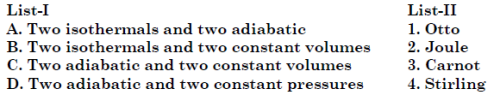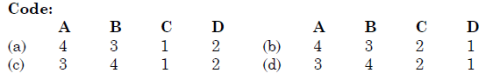Solution:

Ans. (c)

QUESTION: 14

For constant maximum pressure and heat input, the air standard efficiency ofgas power cycles is in the order

Solution:

Ans. (a)

QUESTION: 15

Match List-l (Gas Cycles) with List-ll (Thermodynamic co-ordinates) and select the correct answer using the codes given below the lists: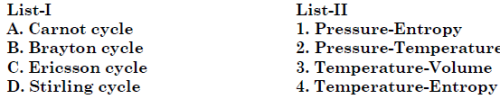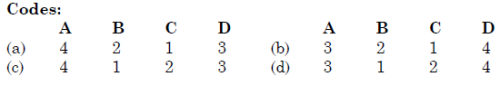Solution:

Ans. (c)

QUESTION: 16

Which one of the following pairs of air standard cycles has the same efficiencyas that of Carnot cycle when operating between the same maximum (source)and minimum (sink) temperatures together with ideal regeneration?

Solution:

Ans. (c)

QUESTION: 17

Pulse jet engine operation can be idealized/ approximated to follow which oneof the following?

Solution:

Ans. (c)

QUESTION: 18

Match List - I (Thermodynamic system/prime mover) with List - II  (Thermodynamic Cycle) and select the correct answer using the codes given below the list: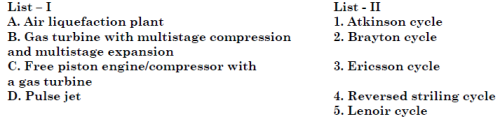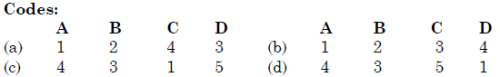Solution:

Ans. (c)

QUESTION: 19

Match List I (Cycle) with List II (Process) and select the correct answer: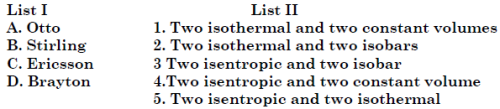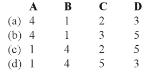Solution:
QUESTION: 20

Which one of the following cycles working within the same temperature limits has the highest work ratio?

Solution:

Ans. (d) Wc is pump work in liquid state i.e. minimum